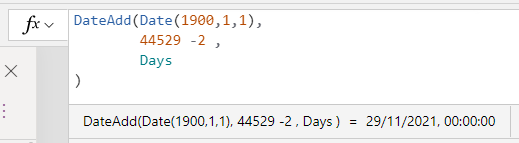# Dates - How to display or convert Excel date serial numbers

Microsoft Excel stores dates as serial numbers. This post describes this behaviour and shows the formula to convert and display Excel date serial numbers in Power Apps.

Internally, Microsoft Excel stores dates as sequential serial numbers. One area where problems can arise is in situations where app builders connect to data sources with records that have been imported from Excel through some other process or workflow automation.

If we find ourselves in a situation where we need to display an Excel date serial number in Power Apps, this post describes the technique we can use.

## What are Excel Serial Numbers?

Excel stores dates as a numeric serial number in order to support differing datetime formats across multiple regions. The official documentation is here.

From Excel, we can retrieve the serial number for a date by calling the DATEVALUE function. The image beneath shows how the serial number for the 29th November 2021 is 44529.The serial number is a representation of the number of days since 1st January 1900. An interesting thing to note is that due to this behaviour, Excel date-related functions don't work for dates prior to 1st January 1900. Prior to this date, Excel stores historical dates as strings. This often causes most problems for historians or genealogy applications. For extra reading, this post by Bill Jelen provides more details.

To also add to the confusion, earlier versions of Excel for Macintosh computers apply a serial number that starts counting from 1st January 1904. Therefore, we may need to adjust the formula in this post in situations where the source data is based on the older 1904 data system.

## How to convert an Excel Date Serial number to a Power Apps date

Let's say we want to convert the serial number 44529 to a date value. We can call the DateAdd function to add the serial number value to 1st January 1900 like so.

`DateAdd(Date(1900,1,1),         44529 -2 ,         Days)`

As the image below highlights, this returns the correct date value.The interesting thing about this formula is how it's necessary to subtract 2 days from the target serial number. What's the reason for this?

The first reason is that serial numbers are 1 based. That is, the serial number for "1st January 1900" is 1, rather than 0. Therefore we need to subtract 1 to account for that.

The second reason is that Excel incorrectly assumes that 1900 is a leap year. The official rule is that every 4th year is a leap year. In addition to this, every 100 years is not a leap year (eg, years 1700, 1800, 1900), but every 400 years is a leap year (eg years 1600, 2000, 2400).  For historic reasons, Excel doesn't correctly apply the 100-year rule, as described in the official documentation.

This means that any serial number beyond 59 (ie 28th February 1900) will be incorrect by 1 day. This accounts for the second day that we need to subtract.

Therefore, in the unlikely event where we want to correctly account for dates before 1st March 1900, the more accurate formula would look like this:
`With({serialNum:60},    DateAdd(Date(1900,1,1),         If(serialNum < 61,serialNum -1,serialNum -2) ,         Days    ))`
The screenshot beneath shows how the formula coerces the serial number of 60 (eg Excel's representation of the non-existent 29th February 1900) to 28th February 1990.## Conclusion

In cases where we need to display Excel date serial numbers, we can carry out this conversion by adding the number to 1st January 1900 and subtracting the value by 2 days (or 1 day where the input date is prior to the 1st March 1900).